# Trigonometry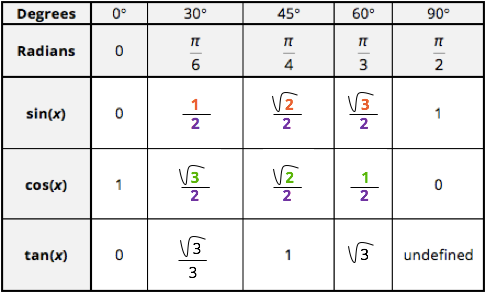## How to remember the exact values of trigonometric functions

Authored By Carmen Popescu-Rose

When you see the table shown below of exact values of the trigonometric functions sin(x), cos(x) and tan(x) you always wonder "How am I going to remember them!?".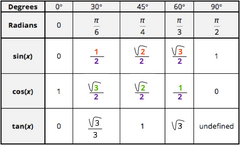Here is an easy way to work them out without having to memorise them.

First of all the sine function increases from 0° to 90°.

sin(0°) = 0 and sin(90°) = 1

Step 1 Write the numbers 1, 2 and 3 from left to right in the empty cells in the sin(x) row as shown below.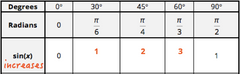Step 2 Square root these three numbers.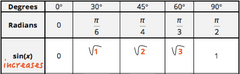Step 3 Draw a fraction line underneath each number.Step 4 Write a 2 at the denominator of each fraction.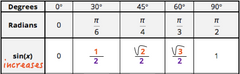... and this is how you can remember the exact values for the sine function from 0° to 90°.

The exact values for the cosine function from 0° to 90° can be worked out in a similar manner.

First of all the cosine function decreases from 0° to 90°.

cos(0°) = 1 and cos(90°) = 0

Step 1 Write the numbers 32 and 1 from left to right in the empty cells in the cos(x) row as shown below.Step 2 Square root these three numbers.Step 3 Draw a fraction line underneath each number.Step 4 Write a 2 at the denominator of each fraction.... and this is how you can remember the exact values for the cosine function from 0° to 90°.

Tags

(0) Items
Items 0
Subtotal \$0.00
To Top